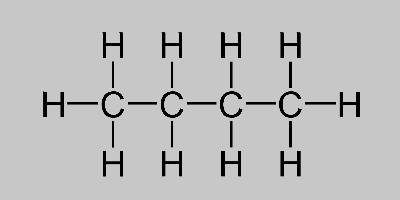# Butane Formula

Understanding the butane formula is important especially when it comes to knowing the mechanism of products that are manufactured in several industries like fuel and refineries amongst others. Butane is an organic compound basically a colourless, odourless and a highly flammable gas. It belongs to the alkane series. Butane is also sometimes referred to as n-butane where its branched chain form is called isobutane.

## Butane Chemical Formula

The formula of butane is C4H10 since the chemical compound is a combination of two hydrocarbons or alkanes where it is further composed of four carbon atoms which are all linked in a straight chain.

 Formula C4H10 Molar Mass 58.124 g·mol-1 Density 2.48 kg/m3 Melting Point −140 to −134 °C Boiling Point −1 to 1 °C

### Butane Structural Formula

Usually, the structure of butane is written as CH3-CH2-CH2-CH3. A representation of the butane structural formula is given below.Learn about all the different formulas of important chemical compounds at BYJU’S.